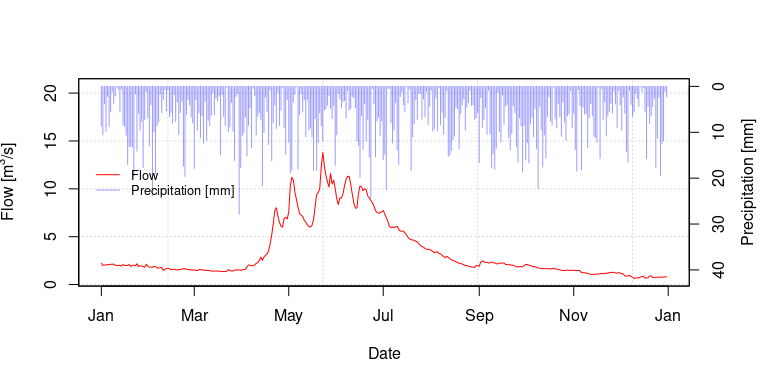# ch_hydrograph_plot

## hydrograph_plot

This is a general-purpose hydrograph plotting function, which is useful for a wide variety of tasks. Currently the function only uses base R plotting, but the addition of ggplot2 graphs is planned for the future. The function can plot any of: observed flows, simulated flows, inflows to a sub-basin, and precipitation on the same graph. The plots can indicate the winter period (which is fixed), and options exist to change the scales and y-axis label.

## Plotting daily streamflows, without and with winter shading

Note that the value returned by the function, if successful, is TRUE

daily_flows <- CAN05AA008[, c(3, 4)]
result1 <- ch_hydrograph_plot(flows = daily_flows, winter_shading = FALSE)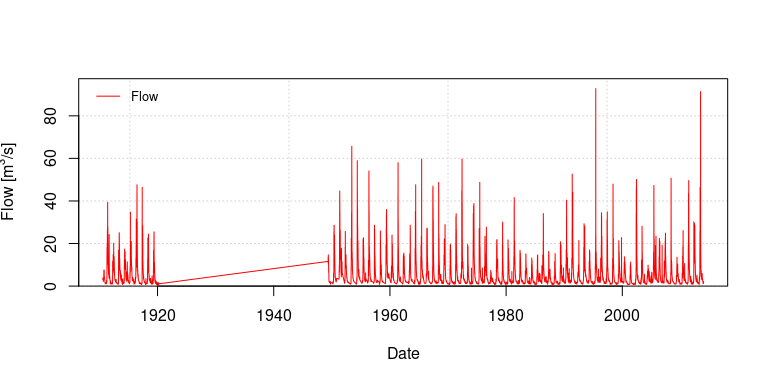result2 <- ch_hydrograph_plot(flows = daily_flows, winter_shading = TRUE)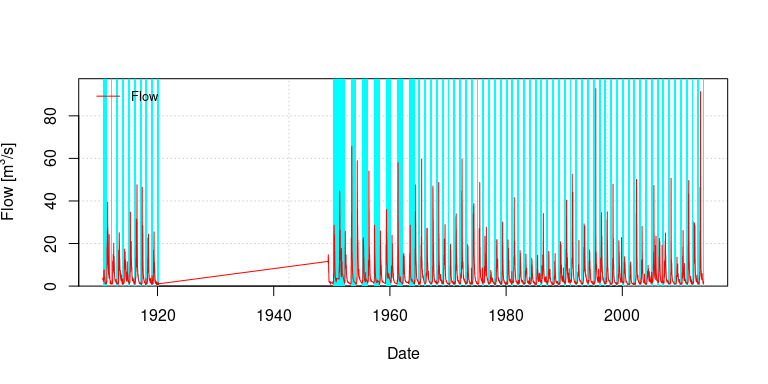The period of the plot can be restricted by setting the option prd, nwhich is a string like “2011-10- 01/2012-09-30” indicating the beginning and end dates of the plot.

myprd <- "2000-01-01/2000-12-31"
result3 <- ch_hydrograph_plot(
flows = daily_flows, winter_shading = TRUE,
prd = myprd
)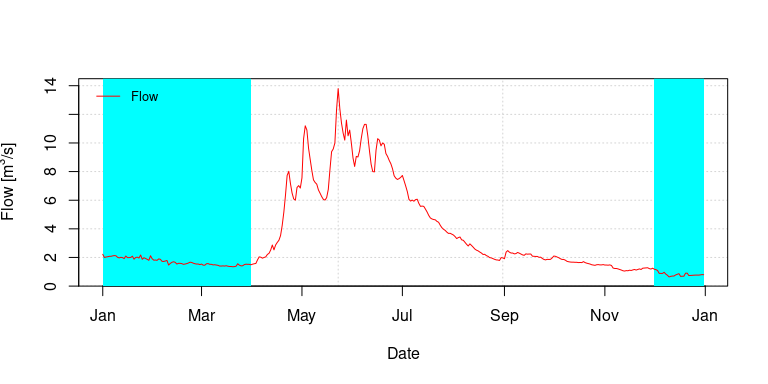You can also plot precipitation data. In this example fake precipitation data are used.

precip <- data.frame("Date" = daily_flows\$Date, "precip" = abs(rnorm(nrow(daily_flows))) * 10)
result4 <- ch_hydrograph_plot(
flows = daily_flows, precip = precip, winter_shading = TRUE,
prd = myprd
)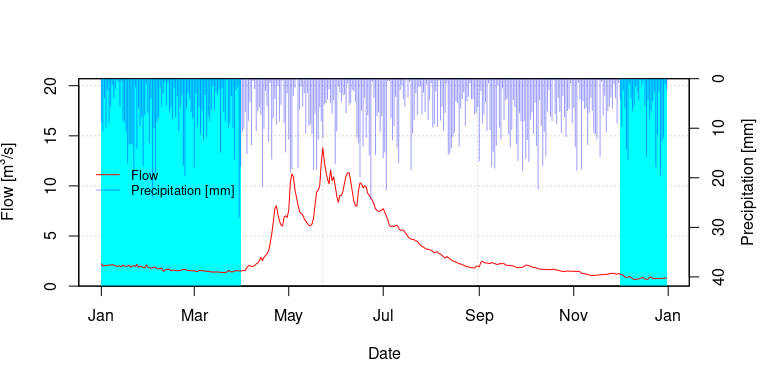The axes of the precipitation and flow can be modified as needed to prevent overlap of the two series, if desired. The default is to multiply the precipitation range by 1.5 of the maximum value, however the range can be multiplied by any positive value to prevent this.

result5 <- ch_hydrograph_plot(
flows = daily_flows, precip = precip, winter_shading = TRUE,
prd = myprd, range_mult_precip = 2, range_mult_flow = 1.8
)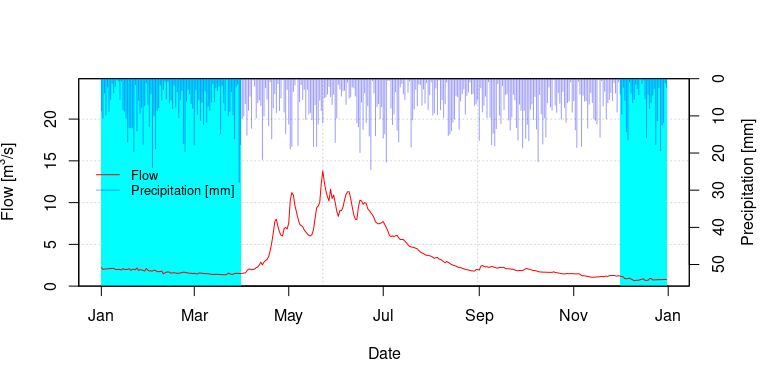## Changing Axis Labels

Only the default y-axis label can be over-ridden. Note that you can use a Unicode character or an expression to get a superscripted 3.

ylab <- expression(paste("Discharge [m"^"3", "/s]"))
result6 <- ch_hydrograph_plot(
flows = daily_flows, precip = precip,
prd = myprd, ylabel = ylab
)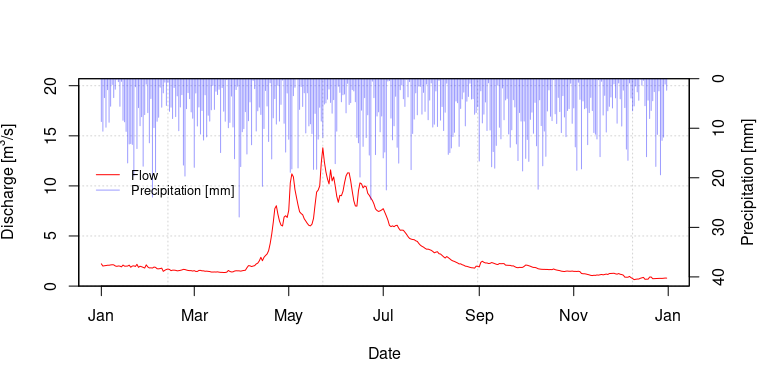The precipitation label can also be adjusted.

ylab_precip <- "Rainfall [mm]"
result7 <- ch_hydrograph_plot(
flows = daily_flows, precip = precip,
prd = myprd, precip_label = ylab_precip
)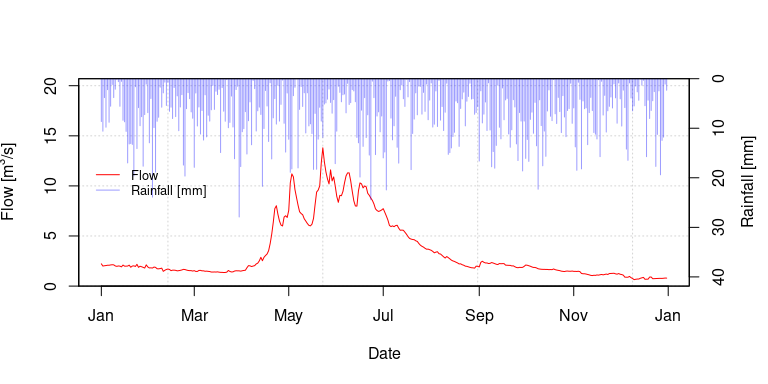## Other format options

Many other formatting options exist, such as:

• changing the legend position
• adding a legend outline and fill to the text box
• forcing the y axis to start at exactly zero

For example:

result8 <- ch_hydrograph_plot(
flows = daily_flows, precip = precip,
prd = myprd, leg_pos = "right"
) # change legend to the right side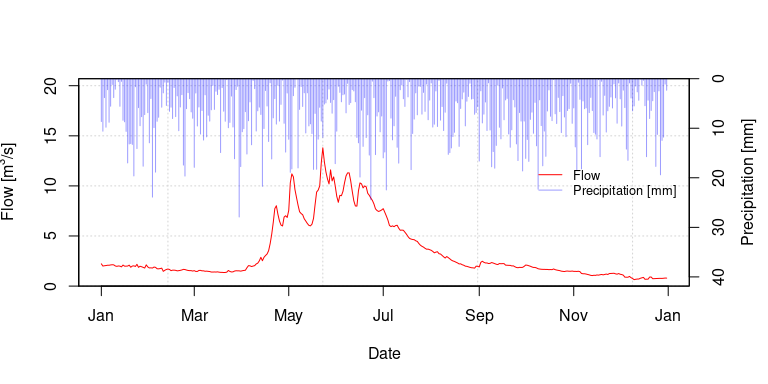result9 <- ch_hydrograph_plot(
flows = daily_flows, precip = precip,
prd = myprd, leg_box = TRUE
) # add legend fill and outline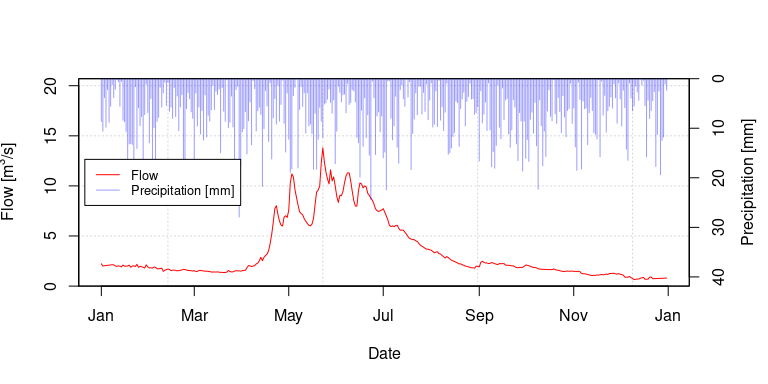result10 <- ch_hydrograph_plot(
flows = daily_flows, precip = precip,
prd = myprd, zero_axis = F
) # default plot outside of function with buffer around axis